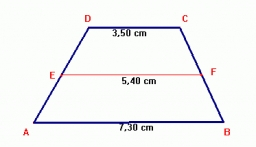# Calculate: 36721

In the trapezoid, it is given:
all four sides a = 51 cm; b = 30 cm; c = 18 cm; d = 27 cm; and height v = 24 cm;
Calculate: perimeter o=? and area S=?

o =  126 cm
S =  828 cm2

### Step-by-step explanation:Did you find an error or inaccuracy? Feel free to write us. Thank you!

#### Grade of the word problem:

We encourage you to watch this tutorial video on this math problem: